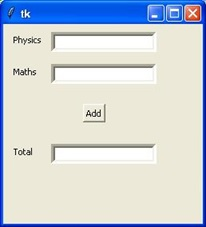# Tkinter place()方法

## 语法

widget.place( place_options )


• anchor - 小部件的确切位置，其他选项参考的位置：可以是N，E，S，W，NE，NW，SE或SW，指示小部件的角落和边缘的罗盘方向；默认为NW（小部件左上角）。

• bordermode - INSIDE（默认值）表示其他选项参考父容器的内部（忽略父容器的边框）；否则为OUTSIDE。

• height, width - 高度和宽度（以像素为单位）。

• relheight, relwidth - 高度和宽度作为介于0.0和1.0之间的浮点数，作为父容器的高度和宽度的一部分。

• relx, rely - 水平和垂直偏移作为介于0.0和1.0之间的浮点数，作为父容器的高度和宽度的一部分。

• x, y - 水平和垂直偏移（以像素为单位）。

## 示例

from tkinter import *
top = Tk()
L1 = Label(top, text="Physics")
L1.place(x=10,y=10)
E1 = Entry(top, bd =5)
E1.place(x=60,y=10)
L2=Label(top,text="Maths")
L2.place(x=10,y=50)
E2=Entry(top,bd=5)
E2.place(x=60,y=50)

L3=Label(top,text="Total")
L3.place(x=10,y=150)
E3=Entry(top,bd=5)
E3.place(x=60,y=150)

B.place(x=100, y=100)
top.geometry("250x250+10+10")
top.mainloop()• 回顶